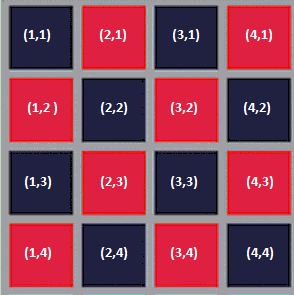# Nested Mappings

## Nested Mapping in Sequences

In the previous subsection, we saw how we can combine enumerate, map, filter, and accumulate to produce more complex functions. In this subsection we are going to explore an example of nested mapping: calling map on a list twice.

## Checker GridJack is a big fan of checkers. He wants to write a function that will make a list all of the coordinates in a 4x4 board. More concretely, he wants a function that outputs:

( (1 . 1) (1 . 2) (1 . 3) (1 . 4)
(2 . 1) (2 . 2) (2 . 3) (2 . 4)
(3 . 1) (3 . 2) (3 . 3) (3 . 4)
(4 . 1) (4 . 2) (4 . 3) (4 . 4) )


Note that a coordinate is represented as a pair of x and y coordinates and the code outputs a list of such coordinates. We will walk step-by-step through how Jack can write this function using list manipulation techniques we have already learned.

## Checker Grid: First RowFirst, lets consider a small chunk of the problem and work our way up: Let's write some code that returns a list of the coordinates from the first row, i.e. ( (1 . 1) (2 . 1) (3 . 1) (4 . 1) ). How can we achieve this? Well, we notice that the x-coordinate starts from 1 and ends at 4 and that the y-coordinate is always 1. So if we have a list (1 2 3 4), we can cons each element with 1. We can write this as:

> (map (lambda (x) (cons x 1))
(enumerate 1 4))
((1 . 1) (2 . 1) (3 . 1) (4 . 1))


So far so good. Jack is happy.

## Checker Grid: All RowsSo we have some code that returns a list of coordinates for the first row. Since there are only 4 rows, we can technically have a copy for each row.

(map    (lambda (x) (cons x **1**))
(enumerate 1 4))

(map    (lambda (x) (cons x **2**))
(enumerate 1 4))

(map    (lambda (x) (cons x **3**))
(enumerate 1 4))

(map    (lambda (x) (cons x **4**))
(enumerate 1 4))


That is good and all, but we know that copying and pasting code is generally a bad idea. (What if the checkerboard was 1000x1000?) We want to keep the part that is similar, and change as little as possible. Notice that the only difference from the code for row1, row2, row3, and row4 is the number you are cons-ing with. We can apply the same method from before:

(map (lambda (y) (map (lambda (x) (cons x y))
(enumerate 1 4)))
(enumerate 1 4))


Notice how the inner lambda takes care of each tile in a single row, while the outer lambda takes care of each row in a board. Hooray! We're done, right?

## Checkers Grid: FlatteningThis is what we get we we run our current code:

> (map (lambda (y)
(map (lambda (x) (cons x y))
(enumerate 1 4)))
(enumerate 1 4))
( ((1 . 1) (2 . 1) (3 . 1) (4 . 1))
((1 . 2) (2 . 2) (3 . 2) (4 . 2))
((1 . 3) (2 . 3) (3 . 3) (4 . 3))
((1 . 4) (2 . 4) (3 . 4) (4 . 4)) )


This looks deceptively similar to our desired result:

( (1 . 1) (2 . 1) (3 . 1) (4 . 1)
(1 . 2) (2 . 2) (3 . 2) (4 . 2)
(1 . 3) (2 . 3) (3 . 3) (4 . 3)
(1 . 4) (2 . 4) (3 . 4) (4 . 4) )


What's different? Our current code returns a list of a list of coordinates. What we want instead is a list of coordinates. So how do we 'flatten' the list? We can call accumulate with append:

(accumulate append
nil
(map (lambda (y)
(map (lambda (x) (cons x y))
(enumerate 1 4))
(enumerate 1 4))


## Flatmap

Calling accumulate with append is so common that we implement this procedure as flatmap:

(define (flatmap proc seq)
(accumulate append nil (map proc seq)))


Using this definition, we can finally write the function that Jack wants:

(flatmap (lambda (y)
(map (lambda (x) (cons x y))
(enumerate 1 4)))
(enumerate 1 4))


## Takeaways

Nested mappings can be useful when you want to traverse a certain list and match their elements up. In order to get your functions correct, breaking the problem down like how we did it here is highly recommended. flatmap is function that "flattens" list of lists.